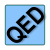Games
Problems
Go Pro!

# Introduction to Proofs

Reference > MathematicsAn explanation of proofs, along with some simple examplesExamples of more algebraic proofs which use additional properties, like the Substitution PropertyUsing definitions of mathematical terminology in proofsAn explanation of theorems, and how we can use them in proofsHow to use the transitive property in proofsHow to prove something by using an assumption that leads to a contradictionIn which we create another algebraic theorem, using two lemmas to help us outAn explanation of corollaries, with some non-geometric examplesMoving beyond two-column proofs to more fluid paragraph style proofsLike us on Facebook to get updates about new resources